Next: Energy Conservation Up: Mathematical Models of Fluid Previous: Momentum Conservation

# Navier-Stokes Equation

Equations (1.24), (1.26), and (1.53) can be combined to give the equation of motion of an isotropic, Newtonian, classical fluid: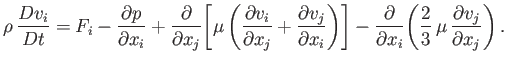(1.54)

This equation is generally known as the Navier-Stokes equation, and is named after Claude-Louis Navier (1785-1836) and George Gabriel Stokes (1819-1903). In situations in which there are no strong temperature gradients in the fluid, it is a good approximation to treat viscosity as a spatially uniform quantity, in which case the Navier-Stokes equation simplifies somewhat to give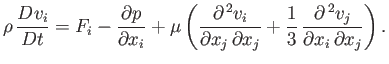(1.55)

When expressed in vector form, the previous expression becomes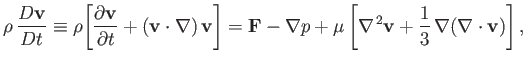(1.56)

where use has been made of Equation (1.39). Here,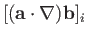(1.57)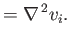(1.58)

Note, however, that the previous identities are only valid in Cartesian coordinates. (See Appendix C.)Next: Energy Conservation Up: Mathematical Models of Fluid Previous: Momentum Conservation
Richard Fitzpatrick 2016-03-31